# Data Types

## Overview

This chapter will introduce VBScript data types, which is closely linked to the subject of Chapter 4, ' Variables and Procedures.' A variable is a name given to a location in memory where some data used by a program is stored. For example, a variable with the name Color might store the value "Blue" . The variable called Color is pointer to a location in the computer's memory where the value "Blue" is stored.

Variables can hold a wide range of data: numbers, dates, text, and other more specialized categories. The different 'categories' into which values can be divided- numbers , dates, text, and so on-are called data types .

Data types help a programming language compiler generate the proper machine instructions from the code that a programmer types in. Even if you did not know a lot about how compilers work, you could imagine that the instructions given to your computer for adding two numbers together are different from the instructions for displaying character text on the screen.

While your success as a VBScript programmer does not depend on your understanding of low-level details such as compilers and machine instructions, it is critical to understand how VBScript handles data types and variables, including the particulars of VBScript's 'universal' data type, the Variant . VBScript has some features and behaviors that are unique and, on the surface, confusing. That is why, even if you are familiar with other programming languages, it is important to read and absorb this chapter.

## Scripting Languages as Loosely Typed

Programming languages come in two flavors when it comes to how data types are handled.

A strongly typed language forces the programmer to declare, in advance, the data type of every variable so that the compiler will know exactly what kind of value to expect in that variable. If the programmer declares a variable with a numeric data type, the compiler expects that variable to hold a number, and produces an error if the programmer violates that assumption by trying to, for instance, store a date in that variable.

In a loosely typed language, the programmer does not have to declare in advance the data type of a variable. Usually, in fact, a loosely typed language does not even provide a way to declare the data type. Scripting languages like VBScript are very often loosely typed. They use a generic, 'universal' super data type that can hold any type of data.

The opposite of a scripting language is a compiled language . Code written in a compiled language is processed in advance by a compiler, which produces an optimized binary executable file-like the .EXE files you are no doubt accustomed to seeing on your computer. A scripting language is not compiled in advance, but rather 'on the fly.' The process for a compiled language is:

1. Write the code in plain text
2. Compile the code to produce an executable file
3. Run the compiled executable file
4. The program runs

Instead of a compiler, most scripting languages, including VBScript, have the concept of a runtime engine , which 'interprets' the code 'at runtime' instead of compiling it in advance. The process for a scripting language goes a bit differently:

1. Write the code in plain text
2. Execute the script file
3. The scripting runtime engine compiles the code 'on the fly'
4. The program runs

The delayed compilation that comes with a scripting language goes hand-in-hand with the loose typing of the language. This is an oversimplification, but since code is compiled on the fly, the compiler can examine the data being placed into a variable and what kinds of operations are being performed on the variable to arrive at an educated guess for what the data type of that variable should be.

The concepts of loose typing, a universal data type, and educated guessing about data types at runtime lead to some interesting scenarios and behaviors when you execute the VBScript code you have written. Throughout this chapter, we will closely examine these details to ensure that you will not fall into any programming traps related to VBScript's unique way of working with variables and data types.

## Why Data Types Are Important

Let's consider for a moment the Visual Basic programmer's perspective on data types. It may seem odd to suddenly change the subject to a different programming language, but VBScript and Visual Basic are actually very closely related and often used together. You can think of Visual Basic as the parent of VBScript. VBScript syntax is derived directly from Visual Basic, and in many cases Visual Basic and VBScript syntax are identical.

However, the reason for bringing up Visual Basic is that, in a discussion of data types, the concepts are simpler to explain and easier to grasp when presented in the context of Visual Basic. What's more, these concepts translate directly when we return in the next section to the discussion of VBScript's Variant data type and its peculiarities .

You will remember from the previous section that Visual Basic is a strongly typed language, which means that a Visual Basic programmer must declare a specific data type for each variable used in his or her program. For example, here is a variable declaration in Visual Basic. What this line of code means is that the programmer is telling the computer to reserve space in memory for a variable called OrderTotal and that the data type that will be stored in that variable is the Currency data type. (The Currency type is used to store numeric values that represent an amount of money.)

```

Dim OrderTotal As Currency

```

By declaring the OrderTotal variable with the Currency data type, the programmer is signaling his or her intention to store only numeric amounts of money in this variable. He does not plan to try to store a date or a customer's name in the OrderTotal variable. And if he did, the Visual Basic compiler would produce an error. Take a look at the next two lines of code, which assign two different values to the OrderTotal variable.

```

OrderTotal = 695.95

OrderTotal = "Bill's Hardware Store"

```

The first line of code works fine, because a numeric value is being stored in the variable. However, the second line of code will produce an error because the type of data going into the variable does not match the declared data type. A strongly typed language also makes a line of code like the following produce an error.

```

OrderTotal = 695.95 + "Bill's Hardware Store"

```

This strongly typed syntax produces several technical benefits in the compilation and performance of a Visual Basic application. However, since this book is about VBScript, we're not going to get into that. What we do want to talk about though are benefits that translate directly to VBScript-namely, the predictability and clarity that strong typing brings to programming.

A programmer always wants to accomplish at least two things: fulfilling the requirements for the program (in other words, building a program that will do what it is supposed to do) and producing a program that is free of bugs and mistakes. Code that is clear, readable, understandable, and predictable will always be easier for human beings to read, understand, and change. Code that is easy to read, understand, and change is always more likely to fulfill the requirements and more likely to be free of bugs than code that is not.

A Visual Basic programmer must declare a variable for a specific purpose, give the variable a specific name, and declare the intention to store only a specific type of data in that variable. If all of the elements of a program are this neatly segmented, given good specific names like OrderTotal , and used in a very consistent manner, the program is likely to do what it's supposed to do without a lot of bugs.

Things are a little different, though, for the VBScript programmer. VBScript does not have any syntax for declaring a variable with the Currency data type, or any other specific data type. All VBScript variables have the same data type, Variant . The following line of code shows what the same variable declaration would look like in VBScript.

```

Dim OrderTotal

```

The syntax is almost the same, but VBScript does not support the As keyword for declaring a data type. This means that the VBScript programmer is free to put any kind of data in this variable he or she wants. The following two lines of VBScript code are both equally valid in VBScript. Unlike in Visual Basic, the second line of code will not produce an error.

```

OrderTotal = 695.95

OrderTotal = "Bill's Hardware Store"

```

Believe it or not, the second line of code that seems so ridiculous does not produce an error in VBScript. As mentioned a moment ago, in Visual Basic this line definitely produces an error when OrderTotal is declared with the Currency data type. However, in VBScript this line of code results in the value "695.95Bill's Hardware Store" stored in the OrderTotal variable.

```

OrderTotal = 695.95 + "Bill's Hardware Store"

```

The reason for these seemingly strange VBScript behaviors will become clear as we dig deeper into the Variant data type and its subtypes . Before we get there, however, there is a lesson to take away from this comparison of Visual Basic and VBScript variables and data types: even though VBScript does not inherently offer the benefits that come with the rigidity of Visual Basic's strong typing and declared data types, VBScript programmers can still realize these benefits. Realizing the benefits takes two things.

First, we must understand how the Variant data type works-in particular, how the Variant subtypes correspond almost exactly to the Visual Basic data types. There are specific ways to control the subtype of a Variant variable so that your programming techniques won't be that much different than if you were programming in Visual Basic. We'll learn these techniques in this chapter.

Second, when we program in VBScript, we must pretend we are programming in Visual Basic. We must pretend that each variable we declare has been declared with a specific data type. Just because the VBScript runtime engine does not care if we store the value "Bob's Hardware Store" in the OrderTotal variable does not mean that we can't be careful to ensure that our code never does that. In fact, when we introduce the 'Hungarian naming convention' later in this chapter you'll see a way that you can declare your intention for each variable to hold a specific data type even though VBScript will not enforce that intention in the way that Visual Basic would.

## The Variant VBScript s Only Data Type

As mentioned in the previous sections, the Variant is the only data type supported in VBScript. Programmers in other nonscripting languages who are accustomed to a wide range of data types that are enforced by a compiler might find this disconcerting. However, the good news is that the Variant is also very flexible. Because the Variant subtype feature allows you to store many different data types and still keep track of what the data type should be, your scripts can handle just about any kind of data you need: numbers , strings (text), and dates, plus other more complex data types such as objects and arrays.

At this point, please flip back to the end of the book and check out Appendix K, 'The Variant Subtypes .' This appendix contains two tables that can be of great use to you as you read along with this chapter and as you write VBScript code on your own.

The first table contains a list of all of the possible subtypes of the Variant data type. For each subtype, you can see the equivalent Visual Basic data type, followed by some information about some special functions that you can use to test for and control what the subtype is in each of your Variant variables . For now, don't worry too much about these function- related columns (we'll get to these very soon). Just take a look at the list of subtypes and how they line up with the Visual Basic data types.

The second table is a list of all of the native Visual Basic data types. As you saw in the first table, all of these data types have an equivalent Variant subtype (that is, except for the Variant data type itself, which is pretty much the same in Visual Basic as it is in VBScript). Take a few moments and look through this second table. Notice what kinds of values can be stored in each of the data types. The properties of each Visual Basic data type are exactly the same as the equivalent Variant subtype.

Keep a bookmark in Appendix K, as you'll want to refer to it as you progress through this chapter.

Returning now to this chapter, let's discuss in more detail the concept of a subtype. A subtype , as the name suggests, is a type within a type. You can think of the Variant itself as the parent data type and the subtype as the child. The parent data type is always Variant , but the child subtype can be one of the many types listed in the aforementioned table in Appendix K. A Variant variable has exactly one subtype; in other words, the Variant 's subtype can only be one type at time. For example, the subtype cannot be both a String and a Long at the same time.

The subtype changes depending on what kind of data your code puts into the variable. As a rule, the subtype and the type of data will always be compatible. For example, it is impossible to have a subtype of Long with the value "Hello" stored in it. If the value of "Hello" was placed into the variable, then the subtype will automatically be String . The Variant will, like a chameleon, automatically change its subtype based on the type of data placed into it. This subtype change process has a fancy name: type coercion .

Even with the fancy name, this subtype concept may seem fairly straightforward. However, there are some real pitfalls waiting for you. And we haven't even brought up implicit versus explicit type coercion. Starting in the next section, we will dig deep into subtypes and type coercion. The investment in reading time (and perhaps trying out the examples) will be well worth it.

### Testing For and Coercing Subtypes

There are two built-in VBScript functions that allow you to check what the subtype is for any Variant variable. These functions are VarType() and TypeName() . These two functions do pretty much the same thing, but VarType() returns a numeric representation of the subtype, and TypeName() returns a text representation. Take a look at the last two columns of the subtypes table in Appendix K, and you'll see the different values that VarType() and TypeName() will return for each of the subtypes. Notice also that there are named constant equivalents for each of the values that VarType() returns.

A named constant is similar to a variable, in that it represents a certain value, but constants cannot be changed at runtime like variables can. You can use a named constant in place of an actual value, which improves the understandability of your code. For example, it's much clearer to write

If VarType(MyVariable) = vbString Then

rather than

If VarType(MyVariable) = 8 Then

VBScript comes with some built-in named constants, and you can also declare your own. We cover constants later in this chapter.

As you can see in the third column of the subtypes table, VBScript also provides some functions that you can use to force (or coerce) the Variant to have a specific subtype, assuming that the value stored in the variable is legal for that subtype. These conversion functions are especially useful when you need to pass data of a certain data type to a VB/COM object that expects data of a specific data type. This is also useful when you want to ensure that the value stored in a Variant variable is treated in a certain way.

For example, the value 12 can be stored in a Variant variable with either a String subtype or one of the numeric subtypes, such as Long . If you want to make sure that the number 12 is treated as a number, and not text, you can use the CLng() conversion function to force the subtype to be Long and not String .

A Variant variable automatically chooses its subtype whenever you place a new value into it. It does this by examining the value placed into it and making its best guess as to what the appropriate subtype is. Sometimes, though, the Variant 's best guess is not quite what you expect. You can control this apparent lack of predictability by being careful and explicit in your code.

Automatic Assignment of String Subtype

Let's look at some code examples that will demonstrate the principles that we have been talking about here.

All of the examples in this chapter are tailored so that they can be run by the Windows Script Host (WSH). The WSH is a scripting host that allows you to run VBScript programs within Windows. WSH will allow you to try out these example programs for yourself. If you are running a newer version of Windows such as Windows 2000 or Windows XP, you should already have the WSH installed. If you are running an older version of Windows, you may or may not have the WSH installed.

To find out, follow the example below by attempting to run the script. To run the script, simply double-click the .VBS file in Windows Explorer. If the script runs, then you're all set. If Windows does not recognize the file, then you'll need to download and install WSH from:

http://msdn.microsoft.com/scripting

Run the script below using the WSH. You can type it in yourself, but it's much easier to download the code for this book from the Wrox Web site. All of the scripts in this chapter are available as individual .VBS files. Throughout the book, before each code example, we will identify the filename in which the script is contained. This script can be found in SUBTYPE_STRING.VBS .

```

Dim varTest

varTest = "Hello There"

MsgBox TypeName(varTest)

```

Running this code results in the dialog box shown in Figure 3-1.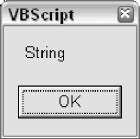Figure 3-1

This makes sense. We placed a text (also known as "String" ) value into the variable varTest , and VBScript automatically decided that the variable should have the String subtype. VBScript Variant variables are smart this way. VBScript takes an educated guess about what the appropriate subtype should be and sets it accordingly . However, the intelligence built into the Variant can also be dangerous, which we'll see as we continue to examine the subtleties of subtypes and type coercion.

Dealing with string values such as "Hello There" is generally straightforward-unless your string value looks like a number, as in the following examples. The script file for the first example is SUBTYPE_STRING2.VBS .

```

Dim varTest

varTest = "12"

MsgBox TypeName(varTest)

```

Running this code results in the exact same dialog box (Figure 3-2) as in the previous example where we used the string "Hello There" .Figure 3-2

Coercing String to Long

At first glance, it may seem like VBScript's Variant is not that smart after all. Why does the TypeName() function return "String" when we clearly passed it a numeric value of 12 ? This is because we placed the value 12 in quotes. VBScript is doing only what we told it to do. By placing the number in quotes, we are telling VBScript to treat the value as a string, not a number. Here are three variations that will tell VBScript that we mean for the value to be treated as a number: SUBTYPE_NUMBER.VBS , SUBTYPE_NUMBER2.VBS , and SUBTYPE_NUMBER3.VBS , respectively.

```

Dim varTest

varTest = 12

MsgBox TypeName(varTest)

Dim varTest

varTest = CInt("12")

MsgBox TypeName(varTest)

Dim varTest

varTest = "12"

varTest = CInt(varTest)

MsgBox TypeName(varTest)

```

All three scripts result in the dialog box shown in Figure 3-3.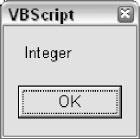Figure 3-3

All these three examples achieve the same thing: coercing the varTest variable to have the Integer subtype. The first example results in the Integer subtype because we did not enclose the value 12 in quotes, as we did previously. Omitting the quotes tells VBScript that we want the number to be treated as a number, not as text.

The second example uses the CInt() conversion function to transform the string value "12" into an integer value before placing it in the variable. This tells the VBScript that we want the subtype to be Integer right from the start.

The third example does basically the same thing as the second but uses two lines of code instead of one. All three examples represent valid ways to make sure that the value we are placing in the variable is treated as a numeric Integer value and not text. However, the first example is better for two reasons: one, because it is more straightforward and succinct; and two, because it is theoretically faster as we're not making the extra call to the CInt() function.

Note that this code would be redundant.

```

Dim varTest

varTest = CInt(12)

```

Because we do not have quotes around the 12 , the subtype will automatically be Integer , and so the CInt() call is unnecessary. However, this code has a different effect.

```

Dim varTest

varTest = CLng(12)

```

This tells VBScript to make sure that the subtype of the variable is Long . The same numeric value of 12 is stored in the variable, but instead of being classified as an Integer , it is classified as a Long . Generally speaking, in a VBScript program this distinction between Integer and Long is not so important, but the distinction would be significant if you were passing the value to a VB/COM function that required a Long . When passing variables between VBScript and VB/COM, it is more important to be particular about data types. (If you remember from the lists of data types earlier in this chapter, Integer and Long are distinguished by the fact that the Long type can hold larger values.)

By default, the Variant subtype will be Integer when a whole number within the Integer range is placed in the variable. However, if you place a whole number outside of this range into the variable, it will choose the Long subtype, which has a much larger range (-2,147,483,648 to 2,147,483,647). You will find that the Long data type is used far more often than the Integer in VB/COM components and ActiveX controls, so you may need to use the CLng() function often to coerce your Variant subtypes to match, although this is not always necessary-when you are passing Variant variables to a COM/VB function, VBScript often takes care of the type coercion for you implicitly (more on this later in the chapter).

Given that VBScript chooses the Integer subtype by default instead of the Long , you would also expect it to choose the Single by default instead of the Double when placing floating-point numbers into a Variant variable, since the Single takes up less resources than the Double . However, this is not the case. When floating-point numbers (that is, numbers with decimal places) are assigned to a Variant variable, the default subtype is Double .

Also, as we'll see later, in the section called Implicit Type Coercion , when you are placing the result of a mathematical expression into an uninitialized Variant variable, VBScript will choose the Double subtype.

Hungarian Notation Variable Prefixes

You may have noticed that we named the variable in the last code examples using the var prefix. This might look strange if you have not seen Hungarian notation before. Hungarian notation is a naming convention for variables that involves the use of prefixes in front of variable names in order to convey the data type of the variable, as well as its 'scope.' (We will discuss scope in the next chapter.)

A data type prefix can tell you the programmer (and other programmers who are reading or modifying your code) what type of data you intend for a variable to hold. In other words, Variant variables can hold any kind of data, but in practice, any given variable should generally hold only one kind of data.

In Visual Basic, since it is a strongly typed language, each variable can hold the type of data only for which it is declared. For example, a Visual Basic variable declared with the Long data type can hold only whole numbers within the lower and upper ranges of the Long data type. In VBScript, however, where every variable is a Variant , any given variable can hold any kind of data.

Remember earlier we said that when we code in VBScript, we want to pretend we are programming in Visual Basic? This is one example of this pretending technique. If we use Hungarian prefixes to signal what kind of data we intend for a variable to hold, it makes it a lot easier to avoid accidentally placing the value "Bill's Hardware Store" in the OrderTotal variable.

Here is a short list of data type prefixes that are commonly used (see Appendix B):

• ar-Variant
• str-String
• int-Integer
• lng-Long
• byt-Byte
• sng-Single
• dbl-Double
• cur-Currency
• obj-Object
• bool-Boolean

The var prefix is best used when you don't know exactly what type of data might end up in the variable, or when you intend for that variable to hold different kinds of data at different times. This is why we're using the var prefix often in this chapter where we're doing all sorts of playing around with data types. In normal practice, however, you will want your variables to have one of the other more specific prefixes listed above or in Appendix B.

Automatic Assignment of the Date Subtype

Let's look at a similar example, this time using date/time values ( SUBTYPE_DATE.VBS ).

```

Dim varTest

varTest = "5/16/99 12:30 PM"

MsgBox TypeName(varTest)

```

Running this code results in the dialog box shown in Figure 3-4.Figure 3-4

The variable assignment results in a subtype of String , although you might expect it to be Date . We get the String subtype because we put the date/time value in quotes. We saw this principle in action in the previous set of examples when we put the number 12 in quotes in the variable assignment. Once again, there are different ways that we can force the subtype to be Date instead of String ( SUBTYPE_DATE2.VBS ).

```

Dim varTest

varTest = #5/16/99 12:30 PM#

MsgBox TypeName(varTest)

```

Or ( SUBTYPE_DATE3.VBS ).

```

Dim varTest

varTest = CDate("5/16/99 12:30 PM")

MsgBox TypeName(varTest)

```

Running either of these examples produces the dialog box shown in Figure 3-5.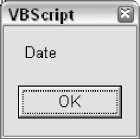Figure 3-5

The first example surrounds the date/time value in # signs instead of quotes. This is the VBScript way of identifying a date literal . A literal is any value that's expressed directly in your code, as opposed to being expressed via a variable or named constant. The number 12 and the string "Hello There" that we used in previous examples are also literals. By enclosing the date/time in # signs rather than quotes, we are telling VBScript to treat the value as a Date , not as a String . As a result, when the Date literal gets stored in the Variant variable, the subtype comes out as Date . The second example uses the CDate() conversion function to achieve the same thing. Once again, the first version is theoretically faster since it does not require an extra function call.

The 'Is' Functions

Often you are not exactly sure what type of data a variable might hold initially, and you need to be sure of what type of data it is before you try to use a conversion function on it. This is important because using a conversion function on the wrong type of data can cause a runtime error. For example, try this code ( SUBTYPE_DATE4_ERROR.VBS ).

```

Dim varTest

varTest = "Hello"

varTest = CLng(varTest)

```

This code will cause a runtime error on line 3: "Type Mismatch" . Not a nice thing to happen when your code is trying to accomplish something. Obviously, this little code sample is pretty silly, because we knew that the variable contained a String when we tried to convert it to a Long . However, you often do not have control over what value ends up in a variable. This is especially true when you are:

• accepting input from the user
• reading data from a database
• reading data from a file

You can often get around these "Type Mismatch" errors by using one of the 'Is' functions that are listed in the fourth column of the Variant subtypes table in Appendix K. For example, here is some code that asks the user his or her age. Since we don't have any control over what the user types in, we need to verify that he or she actually typed in a number ( GET_TEST_AGE.VBS ).

```

Dim lngAge

If IsNumeric(lngAge) Then

lngAge = CLng(lngAge)

lngAge = lngAge + 50

MsgBox "In 50 years, you will be " & CStr(lngAge) & _

" years old."

Else

MsgBox "Sorry, but you did not enter a valid number."

End If

```

Notice how we use the IsNumeric() function to test whether or not the user actually entered a valid number. Since we're planning to use the CLng() function to coerce the subtype, we want to avoid a "Type Mismatch" error. What we have not stated explicitly is that the subtype of the variable does not have to be numeric in order for IsNumeric() to return True . IsNumeric() examines the actual value of the variable, rather than its subtype. The subtype of the variable and the value of the variable are two different things.

This behavior is actually what allows us to use IsNumeric() to avoid a "Type Mismatch" error. If IsNumeric() examined the subtype, it would not be quite so useful. In line 3 of the previous example, the subtype of the lngAge variable is String , yet IsNumeric() returns True if the variable has a number in it. That's because IsNumeric() is considering the value of lngAge , not the subtype. We can test the value before trying to convert the variable's subtype to a different subtype to make sure we don't get that "TypeMismatch" error. This points to a general principle: never trust or make assumptions about data that comes from an external source, in particular from user entry.

The function IsDate() works in exactly the same way as IsNumeric() ( GET_TEST_BIRTH.VBS ).

```

Dim datBirth

datBirth = InputBox("Please enter the date on which " & _

" you were born.")

If IsDate(datBirth) Then

datBirth = CDate(datBirth)

MsgBox "You were born on day " & Day(datBirth) & _

" of month " & Month(datBirth) & " in the year " & _

Year(datBirth) & "."

Else

MsgBox "Sorry, but you did not enter a valid date."

End If

```
 Note Day(), Month(), and Year() are built-in VBScript functions that you can use to return the different parts of a date. These functions are covered in detail in Appendix A.

An exception to the previous statement about the 'Is' functions: not all of the 'Is' functions work strictly on the value, as IsNumeric() and IsDate() do. The functions IsEmpty() , IsNull() , and IsObject() examine the subtype of the variable, not the value. We will cover these three functions later in the chapter.

Before we move on, a brief jump-ahead regarding the use of the If statement in the last code example.

This line of code

```If IsNumeric(lngAge) Then
```

is functionally equivalent to this line

```

If IsNumeric(lngAge) = True Then

```

And likewise, this line

```

If Not IsNumeric(lngAge) Then

```

is functionally equivalent to this line

```

If IsNumeric(lngAge) = False Then

```

However, when using the Not operator, you want to be sure you are using it only in combination with expressions that return the Boolean values True and False (such as the IsNumeric() function). This is because the Not operator can also be used as a 'bitwise' operator (see Appendix A) when used with numeric (non-Boolean) values.

### Implicit Type Coercion

So far, we have been discussing explicit type coercion using conversion functions. We have not yet discussed a phenomenon called implicit type coercion. Explicit type coercion refers to when you the programmer are deliberately changing subtypes using the conversion functions and variable assignment techniques described earlier.

Implicit type coercion is when a Variant variable changes its subtype automatically. Sometimes, implicit type coercion can work in your favor, and sometimes it can present a problem. While this material about type coercion may seem like something you can skip, it is vitally important to understand how this works so that you can avoid hard-to-find bugs in your VBScript programs.

### Implicit Type Coercion in Action

Remember the example code that asks the user for his or her age that we used in the previous section? Here it is again ( GET_TEST_AGE.VBS ).

```

Dim lngAge

If IsNumeric(lngAge) Then

lngAge = CLng(lngAge)

lngAge = lngAge + 50

MsgBox "In 50 years, you will be " & CStr(lngAge) & _

" years old."

Else

MsgBox "Sorry, but you did not enter a valid number."

End If

```

Notice how we use the CLng() and CStr() functions to explicitly coerce the subtypes. Well, in the case of this particular code, these functions are not strictly necessary. The reason is that VBScript's implicit type coercion would have done approximately the same thing for us. Here's the code again, without the conversion functions ( GET_TEST_AGE_IMPLICIT.VBS ).

```

Dim lngAge

If IsNumeric(lngAge) Then

lngAge = lngAge + 50

MsgBox "In 50 years, you will be " & lngAge & _

" years old."

Else

MsgBox "Sorry, but you did not enter a valid number."

End If

```

Because of implicit type coercion, this code works exactly the same way as the original code. Take a look at this line (the fourth line in the above script).

```lngAge = lngAge + 50
```

We did not explicitly coerce the subtype to Long , but the math still works as you'd expect. Let's run this same code, but with some TypeName() functions thrown in so that we can watch the subtypes change ( GET_TEST_AGE_TYPENAME.VBS ).

```

Dim lngAge

MsgBox "TypeName After InputBox: " & TypeName(lngAge)

If IsNumeric(lngAge) Then

lngAge = lngAge + 50

MsgBox "TypeName After Adding 50: " & TypeName(lngAge)

MsgBox "In 50 years, you will be " & lngAge & _

" years old."

Else

MsgBox "Sorry, but you did not enter a valid number."

End If

```

If the user enters, for example, the number 30, this code will result in the dialog boxes, in order, shown in Figures 3-6 to 3-8.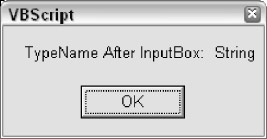Figure 3-6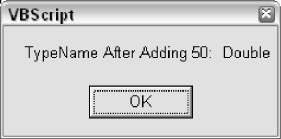Figure 3-7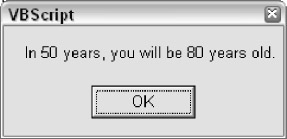Figure 3-8

The first call to the TypeName() function shows that the subtype is String . That's because data coming back from the InputBox() function is always treated as String data, even when the user types in a number. Remember that the String subtype can hold just about any kind of data. However, when numbers and dates (and Boolean True / False values) are stored in a variable with the String subtype, they are not treated as numbers or dates (or as Boolean values)-they are treated simply as strings of text with no special meaning. This is why when our code tries to do math on the String value, VBScript must first coerce the subtype to a numeric one.

It's as if the VBScript compiler behind the scenes is following logic such as this:

1. The variable holds a string value.
2. The code is trying to perform math with the variable.
3. Math requires numeric values. Does the variable hold a numeric value?
4. It does, so do implicit type coercion to change the variable to a numeric subtype.
5. Now that we are sure we are working with a numeric value, do the math the code is asking for.

The second call to the TypeName() function comes after we add 50 to it, and shows that the subtype is Double . Wait a minute- Double ? Why Double ? Why not one of the whole number subtypes, such as Integer or Long ? We didn't introduce any decimal places in this math. Why would VBScript implicitly coerce the subtype into Double ? The answer is because VBScript determined that this was the best thing to do, and since we're trusting VBScript to do the type coercion for us implicitly, we have to accept its sometimes mysterious ways.

Since we did not use a conversion function to explicitly tell VBScript to change the variable to one subtype or another, it evaluated the situation and chose the subtype that it thought was best. VBScript automatically knew that we wanted the value in the variable to be a number. It knew this because our code added 50 to the variable. VBScript says, 'Oh, we're doing some math. I better change the subtype to a numeric one before I do the math, because I can't do math on strings.'

This is pretty straightforward. What isn't so straightforward is that it chose the Double subtype instead of Long or Integer or Byte . This gets to exactly why we're getting into the discussion about implicit type coercion: you have to be careful, because it can be tricky to predict exactly which subtype VBScript will choose.

Before we move one, let's note that there is one other instance of implicit type coercion in our current example. The coercion is incidental, but useful to be aware of. It occurs on this line:

MsgBox "In 50 years, you will be " & lngAge & " years old."

At the time this line executes, we have just finished adding the number 50 to our variable, and the subtype is numeric. When we use the concatenation operator (&) to insert the value of the variable into the sentence , VBScript implicitly changes the subtype to String. This is similar to the way in which the subtype is changed from String to Double when we performed a mathematical operation on it. However, this coercion is not permanent. Since we did not assign a new value to the variable, the subtype does not change.

Avoiding Trouble with Implicit Type Coercion

While you have to be aware of implicit type coercion, there is no reason to fear it. VBScript is not going to arbitrarily go around changing subtypes on its own. There is a logic to what VBScript does. Implicit type coercion only happens when you assign a new value to a variable that does not fit the current subtype. Generally, once a Variant variable has a subtype (based on the value first placed within it, or based on a subtype that your code explicitly coerced), it will keep that subtype as you place new values in the variable.

One way to be sure that implicit type coercion won't cause you any problems is to be careful about using each variable you declare for exactly one purpose. Don't declare generic, multipurpose variables that you use for different reasons throughout your script. If you are going to ask the user for his or her age and then later ask the user for the birth date, don't declare a single generic variable called varInput . Instead, declare two variables, one called lngAge and another called datBirthDate . This makes your code more clear and understandable and helps make sure you don't get in trouble with implicit type coercion.

Where you do need to watch out for implicit type coercion is when you're dealing with a mixture of data types. We saw this in our example: when the data came back from the InputBox() function, it was a string. Then we did some math on it, which turned it into a number. Give this code a try ( IMPLICIT_COERCION.VBS ).

```

Dim lngTest

lngTest = CLng(100)

MsgBox "TypeName after initialization: " & TypeName(lngTest)

lngTest = lngTest + 1000

MsgBox "TypeName after adding 1000: " & TypeName(lngTest)

lngTest = lngTest * 50

MsgBox "TypeName after multiplying by 50: " & _

TypeName(lngTest)

lngTest = "Hello"

MsgBox "TypeName after assigning value of 'Hello': " & _

TypeName(lngTest)

```

If you run this code, you'll see that the first three calls to the TypeName() function reveal that the subtype is Long . Then, after we change the value of the variable to "Hello" , the subtype is automatically coerced into String . What this code illustrates is that once the subtype is established as Long , it stays Long as long as we keep changing the value to other numbers. VBScript has no reason to change it, because the values we put in it remain in the range of the Long subtype. However, when we place text in the variable, VBScript sees that the new value is not appropriate for the Long subtype, and so it changes it to String .

That said, these kinds of mixed-type situations should be rare, and you should try to avoid them. The best way to avoid them is to declare specific variables for specific purposes. Don't mix things up with a single, multipurpose variable like the last code does.

This example reinforces the reason that we use the Hungarian subtype prefix in the variable name. By placing that lng prefix on the variable name, we indicate that we intend for this variable to hold Long numeric values only. The code at the end of our example violates this by changing the value to something nonnumeric. VBScript allows this, because it can't read your mind, but that's not the point.

On the contrary, the fact that the VBScript allows us to store any type of data we please in any variable increases the need for subtype prefixes. The point is to protect our code from strange errors creeping in. Six months from now, if we or someone else were modifying this code, the lng prefix would make it clear that the original intent was for the variable to hold Long numeric values.

In the next example, we will look at how implicit type coercion can happen with numeric variables as the size of the number increases. Give this code a try ( IMPLICIT_COERCION_NUMBER.VBS ).

```

Dim intTest

intTest = CInt(100)

MsgBox "TypeName after initialization to 100: " & _

TypeName(intTest)

intTest = intTest + 1000000

MsgBox "TypeName after adding 1,000,000: " & _

TypeName(intTest)

intTest = intTest + 10000000000

MsgBox "TypeName after adding another 10,000,000,000: " & _

TypeName(intTest)

```

Running this code results in the three dialog boxes shown in Figures 3-9 to 3-11.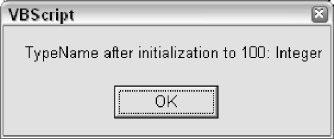Figure 3-9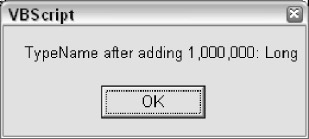Figure 3-10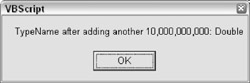Figure 3-11

Notice that we initialize the variable with a value of 100 , and use the CInt() function to coerce the subtype into Integer . The first call to the TypeName() function reflects this. Then we add 1,000,000 to the variable. The next call to the TypeName() function reveals that VBScript coerced the subtype to Long . Why did it do this? Because we exceeded the upper limit of the Integer subtype, which is 32,767. VBScript will promote numeric subtypes when the value exceeds the upper or lower limits of the current numeric subtype. Finally, we add another 10 billion to the variable. This exceeds the upper limit of the Long subtype, so VBScript upgrades the subtype to Double .

Avoiding Trouble with the '&' and '+' Operators

Throughout this chapter you have seen example code that uses the & operator to concatenate strings together. This is a very common operation in VBScript code. VBScript also allows you to use the + operator to concatenate strings. However, this usage of the + operator should be avoided. This is because the + operator, when used to concatenate strings, can cause unwanted implicit type coercion. Try this code ( PLUS_WITH_STRING.VBS ).

```

Dim strFirst

Dim lngSecond

strFirst = CStr(50)

lngSecond = CLng(100)

MsgBox strFirst + lngSecond

```

The resulting dialog box will display the number 150 , which means that it added the two numbers mathematically rather than concatenating them. Now, this is admittedly a very silly example, but it illustrates that the + operator has different effects when you are not using it in a strictly mathematical context. The + operator uses the following rules when deciding what to do:

• If both variables have the String subtype, then VBScript will concatenate them.
• If both variables have any of the numeric subtypes, then VBScript will add them.
• If one of the variables has a numeric subtype, and the other has the String subtype, then VBScript will attempt to add them. If the variable with the String subtype does not contain a number, then a "Type Mismatch" error will occur.

Your best bet is to not worry about these rules and remember only these:

• Use the + operator only when you explicitly want to perform math on numeric values.
• Always use the & operator to concatenate strings.
• Never use the + operator to concatenate strings.

### Empty and Null

You may have noticed that we have not mentioned the first two subtypes in our table of subtypes: Empty and Null . These two subtypes are special in that they do not have a corresponding specific Visual Basic data type. In fact, it's a bit of a misnomer to call these subtypes, because they are actually special values that a Variant variable can hold. When the subtype of a variable is Empty or Null , its value is also either Empty or Null .

This is different from the other subtypes, which describe only the type of value that the variable holds, not the value itself. For example, when the subtype of a variable is Long , the value of the variable can be 0, or 15, or 2,876,456, or one of about 4.3 billion other numbers (-2,147,483,648 to 2,147,483,647). However, when the subtype of a variable is Empty , its value is also always a special value called Empty . In the same fashion, when the subtype of a variable is Null , the value is always a special value called Null .

Empty is a special value that can only be held in a Variant variable. In Visual Basic, variables declared as any of the specific data types cannot hold the value of Empty -only variables declared as Variant can hold the value. In VBScript of course, all variables are Variant variables. A Variant variable is 'empty,' and has the Empty subtype, after it has been declared, but before any value has been placed within it. In other words, Empty is the equivalent of 'not initialized .' Once any type of value has been placed into the variable, it will take on one of the other subtypes, depending on what the value is.

Let's take a look at some examples. First, SUBTYPE_EMPTY.VBS.

```

Dim varTest

MsgBox TypeName(varTest)

```

This simple example results in the dialog box shown in Figure 3-12.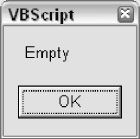Figure 3-12

The subtype is Empty because we have not yet placed any value in it. Empty is both the initial subtype and the initial value of the variable. However, Empty is not a value that you can really do anything with. You can't display it on the screen or print it on paper. It only exists to represent the condition of the variable not having had any value placed in it. Try this code ( SUBTYPE_EMPTY_CONVERT.VBS ).

```

Dim varTest

MsgBox CLng(varTest)

MsgBox CStr(varTest)

```

The code will produce, in succession, the two dialog boxes shown in Figures 3-13 and 3-14.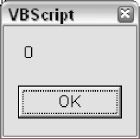Figure 3-13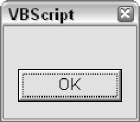Figure 3-14

The first box displays a because Empty is when represented as a number. The second box displays nothing because Empty is an 'empty' or 'zero length' string when represented as a String .

Once you place a value in a Variant variable, it is no longer empty. It will take on another subtype, depending on what type of value you place in it. This is also true when you use a conversion function to coerce the subtype. However, if you need to, you can force the variable to become empty again by using the Empty keyword directly.

```

varTest = Empty

```

You can also test for whether a variable is empty in either of two ways.

```

If varTest = Empty Then

MsgBox "The variable is empty."

End If

```

Or

```

If IsEmpty(varTest) Then

MsgBox "The variable is empty."

End If

```

The IsEmpty() function returns a Variant value of the Boolean subtype with the value of True if the variable is empty, and False if not.

The value/subtype of Null , in a confusing way, is similar to the value/subtype of Empty . The distinction may seem esoteric, but Empty indicates that a variable is uninitialized, whereas Null indicates the absence of valid data. Empty means that no value has been placed into a variable, whereas a Variant variable can only have the value/subtype of Null after the value of Null has been placed into it.

In other words, a variable can only be Null if the Null value has explicitly been placed into it. Null is a special value that is most often encountered in database tables. A column in a database is Null when there is no data in it, and if your code is going to read data from a database, you have to be ready for Null values. Certain functions might also return a Null value.

Another way to think about it is that Empty generally happens by default-it is implicit, because a variable is empty until you place something in it. Null , on the other hand, is explicit-a variable can only be Null if some code made it that way.

The syntax for assigning and testing for Null values is similar to the way the Empty value/subtype works. Here is some code that assigns a Null value to a variable.

```

varTest = Null

```

However, you cannot directly test for the value of Null using the equals ( = ) operator in the same way that you can with Empty -you can use only the IsNull() function to test for a Null value. This is because Null represents invalid data, and when you try to make a direct comparison using invalid data, the result is always invalid data. Try running this code ( NULL_BOOLEAN.VBS ).

```

'This code does not work like you might expect

Dim varTest

varTest = Null

If varTest = Null Then

MsgBox "The variable has a Null value."

End If

```

You did not see any pop-up dialog box here. That's because the expression If varTest = Null always returns False . If you want to know if a variable contains a Null value, you must use the IsNull() function ( NULL_BOOLEAN_ISNULL.VBS ).

```

Dim varTest

varTest = Null

If IsNull(varTest) = True Then

MsgBox "The variable has a Null value."

End If

```

As mentioned, often your code has to be concerned with receiving Null values from a database or a function. The reason we say that you need to be concerned is that, since Null is an indicator of invalid data, Null can cause troubles for you if you pass it to certain functions or try and use it to perform mathematical operations. We saw this just a moment ago when we tried to use the expression If varTest = Null . This unpleasantness occurs in many contexts where you try to mix in Null with valid data. For example, try this code ( NULL_INVALID_ERROR.VBS ).

```

Dim varTest

varTest = Null

varTest = CLng(varTest)

```

Running this code produces an error on line 3: "Invalid Use of Null" . This is a common error with many VBScript functions that don't like Null values to be passed into them. Sometimes, though, you can experience unwanted behavior without an error message to tell you that you did something wrong. Take a look at the odd behavior that results from this code ( NULL_IMPLICIT.VBS ).

```

Dim varTest

Dim lngTest

varTest = Null

lngTest = 2 + varTest

MsgBox TypeName(lngTest)

```

Running this code results in the dialog box shown in Figure 3-15.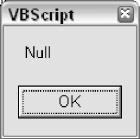Figure 3-15

Did you see what happened here? When we added the number 2 to the value Null , the result was Null . Once again when you mix invalid data ( Null ) with valid data (the number 2 , in this case), you always end up with invalid data.

The following code uses some ADO (ActiveX Data Objects) syntax that you might not be familiar with (see Chapter 18), but here's an example of the type of thing you want to do when you're concerned that a database column might return a Null value.

```

strCustomerName = rsCustomers.Fields("Name").Value

If IsNull(strCustomerName) Then

strCustomerName = ""

End If

```

Here we are assigning the value of the "Name" column in a database table to the variable strCustomerName . If the Name column in the database allows Null values, then we need to be concerned that we might end up with a Null value in our variable. So we use IsNull() to test the value. If IsNull() returns True , then we assign an empty string to the variable instead. Empty strings are much more friendly than Null . This kind of defensive programming is an important technique. Here's a handy shortcut that achieves the same exact thing as the above code.

```

strCustomerName = "" & rsCustomers.Fields("Name").Value

```

Here we are appending an empty string to the value coming from the database. This takes advantage of VBScript's implicit type coercion behavior. Concatenating an empty string with a Null value transforms that value into an empty string, and concatenating an empty string to a valid string has no effect at all, so it's a win-win situation: if the value is Null , it gets fixed, and if it's not Null , it's left alone.

A caution for Visual Basic programmers: you may be accustomed to being able to use the Trim\$ () function to transform Null database values into empty strings. VBScript does not support the '\$' versions of functions such as Trim() , UCase() , and Left() . As you may know, when you don't use the '\$' versions of these functions in Visual Basic, they return a Variant value. This behavior is the same in VBScript, since all functions return Variant values. Therefore, Trim(Null) always returns Null . If you still want to be able to trim database values as you read them in, you need to both append an empty string and use Trim() , like so:

```

strName = Trim("" & rsCustomers.Field("Name").Value)

```

### The Object Subtype

So far, we have not discussed the Object subtype. As the name suggests, a variable will have the Object subtype when it contains a reference to an object. An object is a special construct that contains properties and methods . A property is analogous to a variable, and a method is analogous to a function or procedure. An object is essentially a convenient way of encompassing both data (in the form of properties) and functionality (in the form of methods). Objects are always created at runtime from a class , which is a template from which objects are created (or instantiated ).

For example, you could create a class called Dog . This Dog class could have properties called Color , Breed , and Name , and it could have methods called Bark and Sit . The class definition would have code to implement these properties and methods. Objects created at runtime from the Dog class would be able to set and read the properties and call the methods. A class typically exists as part of a component. For example, you might have a component called Animals that contains a bunch of different classes like Dog , Elephant , and Rhino . The code to create and use a Dog object would look something like this:

```

Dim objMyDog

Set objMyDog = WScript.CreateObject("Animals.Dog")

objDog.Name = "Buddy"

objDog.Breed = "Poodle"

objDog.Color = "Brown"

objDog.Bark

objDog.Sit

```

Don't worry if this is going over your head at this point. We discuss objects and classes in much greater detail throughout the book, starting in Chapter 8. Our point in this section is simply to illustrate how variables with the object subtype behave. Let's look at some code that actually uses a real object: in this case the FileSystemObject , which is part of a collection of objects that allow your VBScript code to interact with the Windows file system. (We discuss FileSystemObject and its cousins in detail in Chapter 7.) The script file for this code is OBJECT_SIMPLE.VBS .

```

Dim objFSO

Dim boolExists

Set objFSO = _

WScript.CreateObject("Scripting.FileSystemObject")

boolExists = objFSO.FileExists("C:autoexec.bat")

MsgBox boolExists

```

In this code, we create a FileSystemObject object and store it in the variable called objFSO . We then use the FileExists function of the object to test for the existence of the autoexec.bat file. Then we display the result of this test in a dialog box. (Note the use of the Set keyword. When changing the value of an object variable, you must use Set .)

Now that you've seen an object in action, let's take a look at two concepts that are germane to this chapter: the IsObject() function, and the special value of Nothing . The script file for this code is OBJECT_ISOBJECT.VBS .

```

Dim objFSO

Dim boolExists

Set objFSO = _

WScript.CreateObject("Scripting.FileSystemObject")

If IsObject(objFSO) Then

boolExists = objFSO.FileExists("C:autoexec.bat")

MsgBox boolExists

End If

```

This illustrates the use of the IsObject() function, which is similar to the other 'Is' functions that we studied earlier in the chapter. If the variable holds a reference to an object (in other words, if the subtype is Object ), then the function will return True . Otherwise, it will return False .

Nothing is a special value that applies only to variables with the Object subtype. An object variable is equal to the value Nothing when the subtype is Object , but the object in the variable either has been destroyed or has not yet been instantiated. The Nothing value is similar to the Null value. When testing for whether an object variable is equal to the value Nothing , you do not use the = operator, as you normally would to test for a specific value. Instead, you have to use the special operator Is . However, when you want to destroy an object, you have to use the Set keyword in combination with the = operator.

If that sounds confusing, don't worry, because it is confusing. Let's look at an example ( OBJECT_SET_ NOTHING.VBS ).

```

Dim objFSO

Dim boolExists

Set objFSO = _

WScript.CreateObject("Scripting.FileSystemObject")

If IsObject(objFSO) Then

boolExists = objFSO.FileExists("C:autoexec.bat")

MsgBox boolExists

Set objFSO = Nothing

If objFSO Is Nothing Then

MsgBox "The object has been destroyed, which " & _

"frees up the resources it was using."

End If

End If

```

Why would you want to destroy an object using the Set < variable > = Nothing syntax? It's a good idea to do this when you are done with using an object, because destroying the object frees up the memory it was taking up. Objects take up a great deal more memory than do normal variables. Also, for reasons too complex to go into here, keeping object variables around longer than necessary can cause fatal memory errors. It's a good idea to develop a habit of setting all object variables equal to Nothing immediately after you are done with them.

### The Error Subtype

We left the Error subtype for last because it is seldom used. However, there's a remote chance that you might end up coming across a component or function that uses the Error subtype to indicate that an error occurred in the function. We are not necessarily endorsing this methodology, but what you might encounter is a function that returns a Variant value that will contain either the result of the function or an error number.

Imagine a function called GetAge() that returns a person's age in years. This function would take a date as a parameter, and return to you the person's age, based on the computer's current system date. If an error occurred in the function, then the return value would instead contain an error number indicating what went wrong. For example:

```

Dim datBirth

Dim lngAge

datBirth = _

InputBox("Please enter the date on which you were born.")

If IsDate(datBirth) Then

lngAge = GetAge(datBirth)

If Not IsError(lngAge) Then

MsgBox "You are " & lngAge & " years old."

Else

If lngAge = 1000 Then

'This error means that the date was greater

'than the current system date.

MsgBox "That date was greater than the " & _

"current system date."

Else

'An unknown error occurred.

MsgBox "The error " & lngAge & _

" occurred in the GetAge() function"

End If

End If

Else

MsgBox "You did not enter a valid date."

End If

```

Keep in mind that GetAge() is a totally fictional function, and you cannot actually run this code (unless you wanted to write a GetAge() function yourself using Visual Basic). The point here is only to illustrate how someone might use the Error subtype, and how your code might have to respond to it. We say might since the Error subtype and the error-returning technique illustrated above is unorthodox and seldom used.

You could not easily implement the use of the Error subtype yourself in VBScript because the VBScript does not support the CVErr() conversion function, as Visual Basic does. (The CVErr() function coerces the subtype of a Variant variable to Error .)

Therefore, without the aid of Visual Basic, you could never coerce the subtype of a variable to be Error . In other words, VBScript code cannot create a variable with the subtype of Error .

There is a 99 % probability that as a VBScript programmer you will never have to worry about the Error subtype.

## Arrays as Complex Data Types

Our discussion so far has focused on variables that hold a single value. However, VBScript can work with two other types of data that are more complex than anything we've looked at so far: objects and arrays. We are not going to discuss objects in this chapter, since they are covered throughout the book, beginning in Chapter 7. However, we are going to take a detailed look at arrays.

### What Is an Array?

An array , as the name suggests, is a matrix of data. While a normal variable has one ' compartment ' in which to store one piece of information, an array has multiple compartments in which to store multiple pieces of information. As you can imagine, this comes in very handy. Even though you might not know it, you are probably already very familiar, outside the context of VBScript, with all sorts of matrices. A spreadsheet is a matrix. It has rows and columns , and you can identify a single 'cell' in the spreadsheet by referring to the row number and column letter where that cell resides. A Bingo game card is also a matrix. It has rows of numbers that span five columns, which are headed by the letters B-I-N-G-O. A database table is a matrix--once again, rows and columns.

An array can be a very simple matrix, with a single column (an array column is called a dimension), or it can be much more complex, with up to 60 dimensions. Arrays are typically used to store repeating instances of the same type of information. For example, suppose your script needs to work with a list of names and phone numbers. An array is perfect for this. Rather than trying to declare separate variables for each of the names and phone numbers in your list (which would be especially challenging if you did not know in advance how many names were going to be in the list), you can store the entire list in one variable.

### Arrays Have Dimensions

A VBScript array can have up to 60 dimensions . Most arrays have either one or two dimensions. A one-dimensional array is best thought of as a list of rows with only one column. A two-dimensional array is a list of values with multiple columns (the first dimension) and rows (the second dimension). Beyond two dimensions, however, the matrix-based rows/columns analogy starts to break down, and the array turns into something much more complex. We're not going to discuss multidimensional arrays much here. Luckily, for the needs of your average script, a two-dimensional array is absolutely sufficient.

Note that a two-dimensional array does not mean that you are limited to two columns. It only means that the array is limited to an x and a y axis. A one-dimensional array really does have two dimensions, but it is limited to a single column. A two-dimensional array can have as many columns and rows as the memory of your computer will allow. For example, here is graphical representation of a one-dimensional array, in the form of a list of colors.

Red

Green

Blue

Yellow

Orange

Black

And here is a two-dimensional array, in the form of a list of names and phone numbers.

Williams

Tony

404-555-6328

Carter

Ron

305-555-2514

Davis

Miles

212-555-5314

Hancock

Herbie

616-555-6943

Shorter

Wayne

853-555-0060

An array with three dimensions is more difficult to represent graphically. Picture a three-dimensional cube, divided up into slices. After three dimensions, it becomes even more difficult to hold a picture of the array's structure in your mind.

### Array Bounds and Declaring Arrays

It's important to make a distinction between the number of dimensions that an array has, and the bounds that an array has. The phone list array above has two dimensions, but it has different upper and lower bounds for each dimension. The upper bound of an array determines how many 'compartments' that dimension can hold. Each of the compartments in an array is called an element . An element can hold exactly one value, but an array can have as many elements as your computer's memory will allow. Here is the phone list array again, but with each of the elements numbered.

1

2

Williams

Tony

404-555-6328

1

Carter

Ron

305-555-2514

2

Davis

Miles

212-555-5314

3

Hancock

Herbie

616-555-6943

4

Shorter

Wayne

853-555-0060

The lower bound of the first dimension (the columns) is , and the upper bound is 2 . The lower bound of the second dimension (the rows) is once again , and the upper bound is 4 . The lower bound of an array in VBScript is always (unlike Visual Basic arrays, which can have any lower bound that you wish to declare). Arrays with a lower bound of are said to be zero based. This can become a bit confusing, because when you are accessing elements in the array, you have to always remember to start counting at , which is not always natural for people. So even though there are three columns in the first dimension, the upper bound is expressed as 2 -because we started numbering them at . Likewise, even though there are five rows in the second dimension, the upper bound is expressed as 4 .

When you declare an array, you can tell VBScript how many dimensions you want, and what the upper bound of each dimension is. There is no need to tell VBScript what you want the lower bound to be because the lower bound is always . For example, here is a declaration for an array variable for the list of colors from the previous section.

```

Dim astrColors(5)

```

The list of colors is one dimensional (that is, it has only one column) and it has six elements. So the upper bound of the array is 5 -remember that we start counting at . Notice the Hungarian prefix (see Appendix B) that we used for our variable name: astr . For a normal string variable name, we would just use the str prefix. We add an extra 'a' in order to convey that this variable is an array. It is very useful for someone reading your code to know that a variable you are using is an array. An additional example: an array of Long numbers would have this prefix- alng . For more information on subtypes and arrays, see the last section of this chapter.

Moving on to the declaration of variables with more than one dimension, here is a declaration for an array variable for our two-dimensional phone list.

```

Dim astrPhoneList(2,4)

```

When we add another dimension, we add a comma and another upper bound definition to the declaration. Since our phone list has three columns, the upper bound of the first dimension is 2 . And since it has five rows, the upper bound of the second dimension is 4 .

Starting in the next section, we're going to cumulatively build a script that will illustrate three things about arrays: how to declare and populate an array; how to add dimensions and elements to an array dynamically; and how to loop through an array and access all of its contents. The variable declaration for astrPhoneList is our first building block. Before we start adding more building blocks, we need to discuss array subscripts.

### Accessing Arrays with Subscripts

In order to read from or write to an array element, you have to use a subscript . A subscript is similar to the column letter and row number syntax that you use in a spreadsheet program. It's also much like the x , y coordinates you learned about in geometry class. Here's our phone list array again, with the elements numbered for convenience.

1

2

Williams

Tony

404-555-6328

1

Carter

Ron

305-555-2514

2

Davis

Miles

212-555-5314

3

Hancock

Herbie

616-555-6943

4

Shorter

Wayne

853-555-0060

The last name "Williams" is stored in subscript 0,0 . The first name "Miles" is stored in subscript 1,2 . The phone number "305-555-2514" is stored in subscript 2,1 . You get the idea.

So now we can add some code that will populate the astrPhoneList array variable with the data for our phone list ( ARRAY_LIST_STATIC.VBS ).

```

Dim astrPhoneList(2,4)

astrPhoneList(0,0) = "Williams"

astrPhoneList(1,0) = "Tony"

astrPhoneList(2,0) = "404-555-6328"

astrPhoneList(0,1) = "Carter"

astrPhoneList(1,1) = "Ron"

astrPhoneList(2,1) = "305-555-2514"

astrPhoneList(0,2) = "Davis"

astrPhoneList(1,2) = "Miles"

astrPhoneList(2,2) = "212-555-5314"

astrPhoneList(0,3) = "Hancock"

astrPhoneList(1,3) = "Herbie"

astrPhoneList(2,3) = "616-555-6943"

astrPhoneList(0,4) = "Shorter"

astrPhoneList(1,4) = "Wayne"

astrPhoneList(2,4) = "853-555-0060"

```

First, this code declares the array variable astrPhoneList . Since we know in advance that we want this array to have three columns (one each for last name, first name, and phone number), and five rows (one for each of the names in our list), we declare the array with the dimensions we want: (2,4) . Then, we add the data to the array, one element/subscript at a time.

When we declared the array variable with the upper bounds (2,4) , VBScript made space in memory for all of the compartments, and the rest of the code puts data into the empty compartments. We use subscripts to identify the compartment we want for each piece of data. We're careful to be consistent by making sure that last names, first names, and phone numbers each go into the same column across all five rows.

But what happens when we don't know in advance how many elements we're going to need in our array? This is where the dynamic array comes in. A dynamic array is one that is not preconstrained to have certain upper bounds, or even a certain number of dimensions. You can declare the array variable once at design time, then change the number of dimensions and the upper bound of those dimensions dynamically at runtime. In order to declare a variable as a dynamic array, you just use the parentheses without putting any dimensions in them.

```

Dim astrPhoneList()

```

The parentheses after the variable name tells VBScript that we want this variable to be an array, but the omission of the upper bounds signals that we don't know at design time how many elements we're going to need to store in it. This is a very common occurrence-perhaps more common than knowing in advance how many elements you're going to need.

If you're going to open a file or database table and feed the contents into an array, you might not know at design time how many items will be in the file or database table. Since the number of columns in a database table is relatively fixed, you can safely hard-code an assumption about the number of columns. However, you would not want to assume how many rows are in the table. The number of rows in a database table can potentially change frequently. Even if you know how many rows there are right at this moment, you would not want to hard-code that assumption. So the dynamic array solves that dilemma by allowing us to resize the array at runtime.

In order to change the number of dimensions in a dynamic array you have to use the ReDim statement. You can use the ReDim statement anywhere in any code that is in the same scope as the dynamic array variable (for more about 'scope,' see Chapter 4).

However, there is one caveat to keep in mind with ReDim : using ReDim all by itself clears out the array at the same time that it resizes it. If you stored some data in the array, and then used ReDim to resize it, all the data you previously stored in the array would be lost. Sometimes that's a good thing, sometimes it's not-it depends on the program you're writing. In those cases where you don't want to lose the data in the array as you resize it you want to use the Preserve keyword. Using the Preserve keyword ensures that the data you've already stored in the array stays there when you resize it. (However, if you make the array smaller than it already was, you will of course lose the data that was in the elements you chopped off, even if you use the Preserve keyword.)

Below is our phone list code, this time with two changes. First, we've changed the declaration of the array variable so that it is a dynamic array. Second, we've changed the code that populates the array so that it uses the ReDim statement with the Preserve keyword to add rows to the array as we go ( ARRAY_LIST_DYNAMIC.VBS ).

```

Dim astrPhoneList()

ReDim Preserve astrPhoneList(2,0)

astrPhoneList(0, 0) = "Williams"

astrPhoneList(1, 0) = "Tony"

astrPhoneList(2, 0) = "404-555-6328"

ReDim Preserve astrPhoneList(2,1)

astrPhoneList(0, 1) = "Carter"

astrPhoneList(1, 1) = "Ron"

astrPhoneList(2, 1) = "305-555-2514"

ReDim Preserve astrPhoneList(2,2)

astrPhoneList(0, 2) = "Davis"

astrPhoneList(1, 2) = "Miles"

astrPhoneList(2, 2) = "212-555-5314"

ReDim Preserve astrPhoneList(2,3)

astrPhoneList(0, 3) = "Hancock"

astrPhoneList(1, 3) = "Herbie"

astrPhoneList(2, 3) = "616-555-6943"

ReDim Preserve astrPhoneList(2,4)

astrPhoneList(0, 4) = "Shorter"

astrPhoneList(1, 4) = "Wayne"

astrPhoneList(2, 4) = "853-555-0060"

```

There is one caveat when using the Preserve keyword: you can only resize the last dimension in the array. If you attempt to resize any dimension other than the last dimension, VBScript will generate a runtime error. That's why, when working with two-dimensional arrays, it's best to think of the first dimension as the columns, and the second dimension as the rows. You will generally know how many columns you need in an array at design time, so you won't have to resize the columns dimension.

It's the number of rows that you generally won't be sure about. For example, in our phone list array, we know that we need three columns: one for the last name, one for the first name, and one for the phone number. So we can hard code these at design time and dynamically resize the rows dimension at runtime. Regardless, make sure that the dimension you want to resize with ReDim Preserve is the last dimension in your array.

Note that when you declare a variable with the parentheses at the end of the variable name-for example, varTest() -that variable can only be used as an array. However, you can declare a variable without the parentheses at the end, and still use the ReDim statement later to turn it into a dynamic array. Then you can assign a normal number to the variable again to stop it from being an array. However, using a variable for multiple purposes in this manner can be confusing and might allow bugs to creep into your code. If you need a variable to be both an array and not an array, you might consider declaring two separate variables instead of using one variable for two purposes.

### Looping through Arrays

Now that we've declared an array, sized it appropriately, and filled it up with data, let's do something useful with it. Below is our code, this time with some new additions. We've added a few more variables, and we've added a block of code at the end that loops through the array and displays the contents of the phone list ( ARRAY_LIST_DISPLAY.VBS ).

```

Dim astrPhoneList()

Dim strMsg

Dim lngIndex

Dim lngUBound

ReDim Preserve astrPhoneList(2,0)

astrPhoneList(0, 0) = "Williams"

astrPhoneList(1, 0) = "Tony"

astrPhoneList(2, 0) = "404-555-6328"

ReDim Preserve astrPhoneList(2,1)

astrPhoneList(0, 1) = "Carter"

astrPhoneList(1, 1) = "Ron"

astrPhoneList(2, 1) = "305-555-2514"

ReDim Preserve astrPhoneList(2,2)

astrPhoneList(0, 2) = "Davis"

astrPhoneList(1, 2) = "Miles"

astrPhoneList(2, 2) = "212-555-5314"

ReDim Preserve astrPhoneList(2,3)

astrPhoneList(0, 3) = "Hancock"

astrPhoneList(1, 3) = "Herbie"

astrPhoneList(2, 3) = "616-555-6943"

ReDim Preserve astrPhoneList(2,4)

astrPhoneList(0, 4) = "Shorter"

astrPhoneList(1, 4) = "Wayne"

astrPhoneList(2, 4) = "853-555-0060"

'Loop through the array and display its contents

lngUBound = UBound(astrPhoneList, 2)

strMsg = "The phone list is:" & vbNewLine & vbNewLine

For lngIndex = 0 to lngUBound

strMsg = strMsg & astrPhoneList(0, lngIndex) & ", "

strMsg = strMsg & astrPhoneList(1, lngIndex) & " - "

strMsg = strMsg & astrPhoneList(2, lngIndex) & vbNewLine

Next

MsgBox strMsg

```

Running this script results in the dialog box shown in Figure 3-16.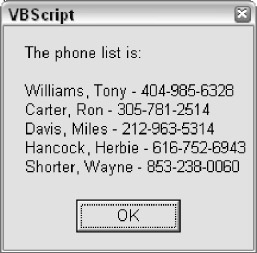Figure 3-16

Let's examine the additions to our code. First, we've added three new variables.

```Dim strMsg
Dim lngIndex
Dim lngUBound
```

These are used in the new block of code at the end of the script. The strMsg variable stores the text version of the phone list that we build dynamically as we loop through the array. The lngIndex variable is used to keep track of which row we are on inside the loop. Finally, lngUBound is used to store the count of rows in the array.

Turning our attention to the new block of code, first we use the UBound() function to read how many rows are in our array.

```lngUBound = UBound(astrPhoneList, 2)
```

The UBound() function is very useful in this type of situation because it keeps us from having to hard-code in our loop an assumption about how many rows the array has. For example, if we added a sixth row to the array, the loop-and-display code would not need to change at all because we used the UBound() function to keep from assuming the number of rows.

The UBound() function takes two arguments. The first argument is the array variable that you wish the function to measure. The second argument is the number for the dimension you wish to have a count on. In our code, we passed in the number 2 , indicating the second dimension-that is, the rows in the phone list array. If we had wanted to count the number of columns, we would have passed the number 1 . Notice that this argument is 1 based, not based. This is a little confusing, but that's the way it is.

Next we initialize the strMsg variable.

```strMsg = "The phone list is:" & vbNewLine& vbNewLine
```

As we go through the loop, we continually append to the end of this variable, until we have a string of text that we can feed to the MsgBox() function. We initialize it before we start the loop. vbNewLine is a special named constant that is built into VBScript that you can use whenever you want to add a line break to a string of text. (You can learn more about named constants and why they're so important in Chapter 4.)

Next we have our loop.

```For lngIndex = 0 to lngUBound
strMsg = strMsg & astrPhoneList(0, lngIndex) & ", "
strMsg = strMsg & astrPhoneList(1, lngIndex) & " - "
strMsg = strMsg & astrPhoneList(2, lngIndex) & vbNewLine
Next
```

We're going to ignore for now the exact syntax of the loop, since loop structure and syntax is covered in detail in Chapter 5. If the syntax is unfamiliar to you, don't worry about that for now. Let's point out a few things, though. First, notice that we are using the lngUBound variable to control how many times we go through our loop.

Second, notice that the lngIndex variable automatically increases by 1 each time we go through the loop. It starts out at , and then for each row in the array, it increases by 1 . This allows us to use lngIndex for the row subscript when we read from each element of the array. This illustrates another good thing to know about array subscripts: you don't have to use literal numbers as we had been in all of our previous examples; you can use variables as well.

Finally, when the loop is done, we display the phone list in the dialog box shown in Figure 3-16.

```MsgBox strMsg
```

### Erasing Arrays

You can totally empty out an array using the Erase statement. The Erase statement has slightly different effects with fixed size and dynamic arrays. With a fixed size array, the data in the array elements is deleted, but the elements themselves stay there-they're just empty. With a dynamic array, the Erase statement completely releases the memory the array was taking up. The data in the array is deleted, and the elements themselves are destroyed . To get them back, you would have to use the ReDim statement on the array variable again. Here's an example.

```

Erase astrPhoneList

```

### Using VarType() with Arrays

The Microsoft VBScript documentation has an error in its description of the VarType() function in regards to arrays. It states that when you use the VarType() function to determine the subtype of an array variable, the number returned will be a combination of the number 8192 and the normal VarType() return value for the subtype (see the table in Appendix K for a list of all the subtype return values and their named constant equivalents). The named constant equivalent for 8192 is vbArray .

According to the documentation, you can subtract 8192 from the VarType() return value to determine that actual subtype. This is only partially correct. The VarType() function does indeed return 8192 ( vbArray ) plus another subtype number-but that other subtype number will always be 12 ( vbVariant ). The subtype of a VBScript array can never be anything but Variant .

Give this code a try and you'll see that no matter what types of values you try to place in the array ( String , Date , Long , Integer , Boolean , and so on), you'll never get the message box in the Else clause to display ( ARRAY_VARTYPE_NOMSG.VBS ).

```

Dim strTest(1)

Dim lngSubType

strTest(0) = CLng(12)

strTest(1) = "Hello"

lngSubType = VarType(strTest) - vbArray

If lngSubType = vbVariant Then

MsgBox "The Subtype is Variant."

Else

MsgBox "The subtype is: " & lngSubType

End If

```

A final note for Visual Basic developers: since we are discussing complex data types, keep in mind that User Defined Types (UDTs) are not supported in VBScript. You cannot define UDTs with the Type statement, nor can you work with UDT variables exposed by VB components .

## Summary

In this chapter we covered the ins and outs of VBScript data types, including one of the 'complex' data types, arrays. VBScript supports only one data type, the Variant , but the Variant data type supports many ' subtypes .'

A Variant variable always has exactly one subtype. Subtypes are determined either implicitly or explicitly. Implicit setting of the subtype occurs when you assign a value to a variable. Sometimes VBScript will change the subtype of a variable 'behind your back' without your realizing it. It is important to understand when and why VBScript implicitly coerces subtypes. Sometimes you can use implicit type coercion to your advantage. In addition, as a VBScript programmer you can explicitly set the subtype of a variable using conversion functions such as CLng() .

You can test for the subtype of a Variant variable using functions such as IsNumeric() and IsNull() . In addition, you can obtain the name of a variable's subtype using the VarType() function. Often it is important to test the subtype rather than making assumptions about it. Errors and unwanted behavior can result in certain circumstances if you are not careful with the subtype of your variables .

VBScript also has some 'special' subtypes such as Empty , Null , and Object , and it is important for a VBScript programmer to understand these subtypes.

In this chapter we also covered arrays, which, along with objects, are a form of complex data type. An array is considered 'complex' because it can hold many values at the same time. Arrays hold multiple values in a 'dimensional' structure. Most commonly, arrays are two dimensional, much like a grid or database table. However, arrays can also have more than two dimensions. Data can be placed into and read from arrays using 'subscripts,' which is a convention for referring to a particular location within the dimensional array structure.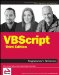VBScript Programmers Reference
ISBN: 0470168080
EAN: 2147483647
Year: 2003
Pages: 242